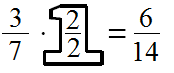### Home > MC2 > Chapter 3 > Lesson 3.1.2 > Problem3-22

3-22.

Simplify the following expressions.

1. $\frac { 2 } { 5 } - \frac { 1 } { 6 }$

You must find a common denominator before you can combine these terms.

A common denominator is often a multiple of both denominators of both fractions that are to be combined. A good one to use for this expression would be $30$.

1. $\frac { 3 } { 7 } - \frac { 7 } { 14 }$Now combine and simplify the entire expression.

1. $\frac { 5 } { 8 } - \frac { 2 } { 3 }$

Refer to part (a).